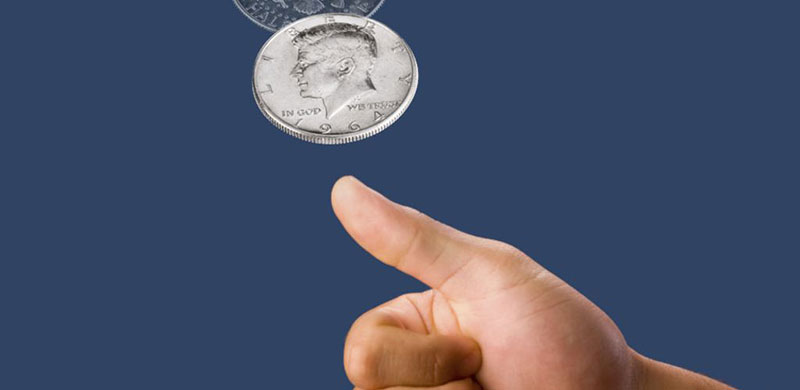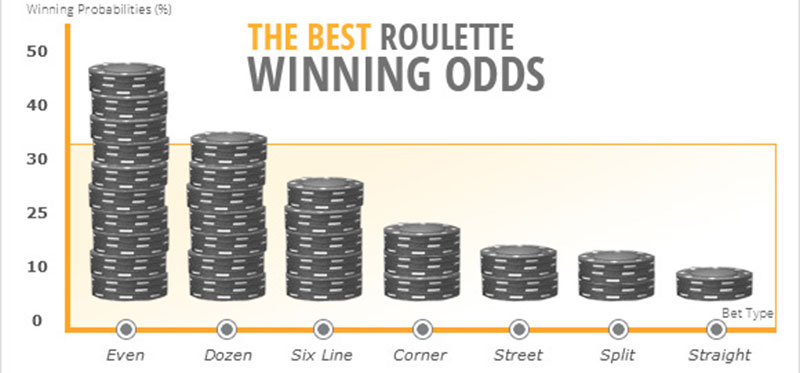# Odds and ProbabilitiesOnce players have become acquainted with the different types of bets they can place in roulette, they can turn their attention to the mathematical side of the game. Many casual players ignore this aspect of roulette. However, prior to placing real-money wagers, it is advisable to get a better idea about the odds and probabilities of winning with different types of bets. This will enable players to balance the risks and their potential profits and help them implement a suitable betting strategy to minimise their losses.

Many inexperienced roulette players fail to make a distinction between the concepts of odds and probabilities. Yet, a certain difference exists. Read on to get a better idea what the two terms mean and how you can calculate both the odds and the probabilities of winning with each type of bet in roulette.

## What Does the Term “Probability” Mean?

Generally speaking, the term “probability” can be defined as the likelihood that a specific event or outcome will occur. In casino games like roulette, however, the term denotes the likelihood that a given number will come up. When the concept of probability is applied to roulette, it is expressed as “1 out of 37”, for example, or is written down as 1/37, which is the same thing.

When the probability is expressed as a fraction, the first number stands for the expected frequency of a given event occurring. The second number or 37 in our example, represents the overall number of possible outcomes. In European roulette, all 37 numbers have equal chances of coming up. For every straight up bet, there are two possible outcomes – the player either wins or loses. So in this instance, 1 represents the number of ways to win with a straight up bet, while 37 stands for the total number of possible outcomes.

The concept of probability can best be explained by giving the coin-flip as an example. Ask yourselves what is the probability of tails coming up the next time you flip the coin. There are only two possible outcomes of a coin flip – either heads or tails will come up.

In this instance, we are betting that tails will come up so the probability of this happening can be expressed as 1 out of 2, 1 to 2, 1:2 or 1 / 2. To convert the probability of tails coming up into a percentage, one needs to divide the first number of the fraction by the second number. This way we get the following result: 1 / 2 = 0.50 x 100 = 50%. Bear in mind the total number of outcomes includes both the ways to win and the possible ways to lose.### Calculating Probability in Roulette

Naturally, this principle can be applied in the game of roulette as well. Let us assume we are playing the European variation of the game where there are 36 numbered pockets on the wheel plus a single-zero pocket. This makes for a total of 37 possible outcomes on any given spin of the wheel. You want to place a straight up bet on an individual number, number 16, for example. But before you put your money on stake, you want to figure out what the probability of number 16 coming up on the next spin of the wheel is.

A seasoned gambler would say the probability of 16 winning is 1 to 37. And indeed it is, but we want to convert it to a percentage. We divide the number of ways to win by the number of all possible outcomes and get the following: 1 / 37 = 0.0270. Then, we multiply the decimal number by 100 and the result is: 0.0270 x 100 = 2.70. It turns out the probability of you winning with a straight up bet on number 16 is 2.70%.

The formula can be used to calculate the probability of winning of all roulette bets. Let’s provide another example with an even-money bet. Assume you want to place an even-money bet on Red. As we know, there are 18 red and 18 black pockets on the wheel, plus the single-zero pocket. This makes for 18 ways to win out of 37 possible outcomes. The calculation will go as follows: 18 / 37 = 0.4864 x 100 = 48.64%. Many inexperienced players wrongfully assume they have equal chances of winning and losing with even-money bets, but apparently this is not so. The reason lies in the single-zero pocket which gives the house its edge.

Let us finish with one last example and assume you wish to place a corner bet on numbers 7, 8, 10 and 11. This way, you will have 4 ways to win out of 37 possible outcomes. The calculation in this case will be: 4 / 37 = 0.1081 x 100 = 10.81%. Not too shabby, especially when compared to your chances of winning with a straight up bet.

European Roulette House Edge and Payout
Bet Type Bet Payout Bet Probability House Edge
Straight 35/1 2.70% 2.70%
Split 17/1 5.41% 2.70%
Street 11/1 8.11% 2.70%
Square or Corner 8/1 10.81% 2.70%
Six Line 5/1 16.2% 2.70%
Column 2/1 32.4% 2.70%
Dozen 2/1 32.4% 2.70%
Red / Black 1/1 48.64% 2.70%
Odd / Even 1/1 48.64% 2.70%
High / Low 1/1 48.64% 2.70%
American Roulette House Edge and Payout
Bet Type Bet Payout Bet Probability House Edge
Straight 35/1 2.63% 5.26%
Split 17/1 5.26% 5.26%
Street 11/1 7.89% 5.26%
Square or Corner 8/1 10.53% 5.26%
Five Line 6/1 13.16% 7.89%
Six Line 5/1 15.79% 5.26%
Column 2/1 31.58% 5.26%
Dozen 2/1 31.58% 5.26%
Red / Black 1/1 46.37% 5.26%
Odd / Even 1/1 46.37% 5.26%
High / Low 1/1 46.37% 5.26%
French Roulette House Edge and Payout *
Bet Type Bet Payout Bet Probability House Edge
Straight 35/1 2.70% 2.70%
Split 17/1 5.41% 2.70%
Street 11/1 8.11% 2.70%
Trio (0,1,2 / 0,2,3) 11/1 8.11% 2.70%
Four-Number (0,1,2,3) 8/1 10.81% 2.70%
Square or Corner 8/1 10.81% 2.70%
Six Line 5/1 16.2% 2.70%
Column 2/1 32.4% 2.70%
Dozen (P12, M12, D12) 2/1 32.4% 2.70%
Red / Black 1/1 48.64% 2.70%
Impair (Odd) / Pair (Even) 1/1 48.64% 2.70%
Manque (Low) / Passe (High) 1/1 48.64% 2.70%

* In French roulette, when even money bets are placed and the ball ends up in the zero pocket, only half of the bet is returned to the player. This is known as the “half-black” or “en prison” rule and reduces the house edge for even money bets to 1.35%.

## What Does the Term “Odds” Mean?

Casual players make the mistake to assume “odds” and “probabilities” are two interchangeable terms. They are not. The term “odds” refers to the likelihood of a specific outcome to occur compared to the likelihood of the same outcome not occurring.

Similarly to probabilities, odds, too, can be expressed as two numbers in a specific ratio. In this case, the first number stands for the expected frequency of a given outcome occurring. The second number represents the number of the remaining possible outcomes and excludes that first specific outcome.

Let us now go back to our coin-flip example. This time, you decide to bet on heads and the only other option you have is tails. The odds of heads coming up the next time you flip the coin can be expressed as 1 to 1, 1:1 or 1/1. One important thing to remember is that probabilities can be expressed as both percentages and ratios, while odds are only expressed as ratios.### Calculating the Odds

Now, let us return to the game of European roulette. You are still considering to place a straight up bet on the number 16 and are wondering what are the odds of this particular number showing up when the ball loses momentum and comes to a stop.

With straight up bets there is only 1 way to win and 36 ways to lose. So, the odds of your number coming up can be expressed as 1 to 36 or 1:36. It is worth mentioning the odds can be represented in reverse, for example 36:1, which is confusing to some. Nevertheless, the ratio still indicates there are 36 ways to lose with a straight bet and only one way to win. Similarly, the odds of winning with an even-money bet are represented by the ratio 18:19, while the odds of losing can be shown as 19:18.

It is important to mention these are the true odds which represent the actual possibility of winning and losing with your bets in roulette. However, the casino will not pay off your winnings on the basis of the true odds. Winning straight up bets are paid off at odds of 1:35, while even-money wagers are paid out at odds of 1:1 (or 18:18) even though players’ chances of winning and losing are not 100% equal because of the single-zero pocket on the European roulette wheel. To calculate the house edge, players can divide the number of ways to win by the overall number of possible outcomes. In this case the result will be the following: 1 / 37 = 0.0270 x 100 = 2.70%.

In European roulette, the house has one unit of profit, while in American roulette where there are 38 pockets, the casino collects two units of profit per each winning straight up bet.